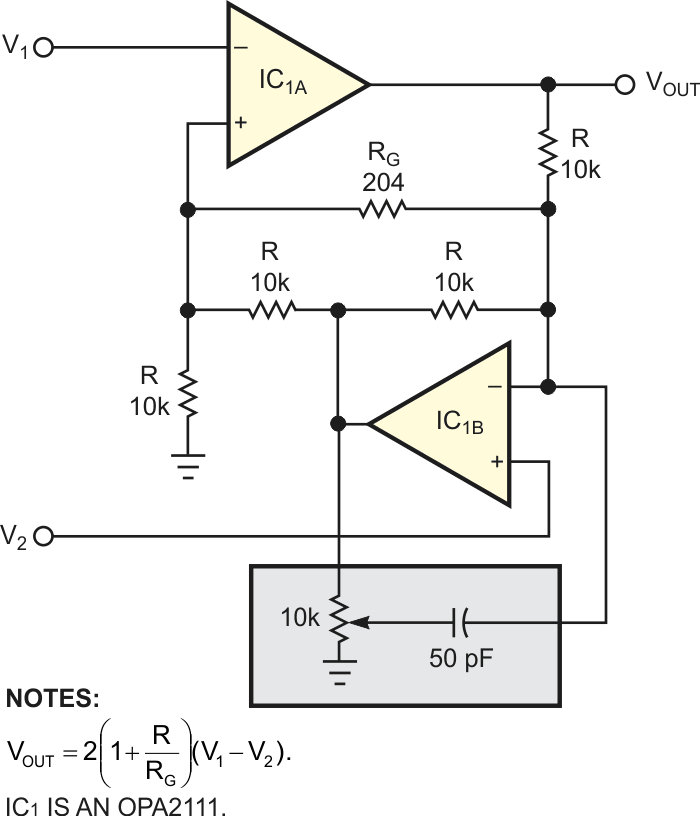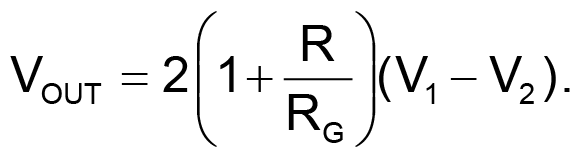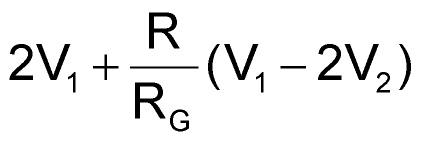# Use Dual Op Amp in an Instrumentation Amp

## Texas Instruments OPA2111

Although monolithic instrumentation amplifiers are more cost-effective than their discrete and modular predecessors, the limited variety of monolithic instrumentation amps restricts their use. You can widen your options, however, by deriving the differential response of an instrumentation amplifier from a dual op amp (Figure 1). The circuit uses FET-input op amps to provide lower noise and lower input-bias currents than monolithic instrumentation amps can offer.Figure 1. You can build an instrumentation amplifier by providing a common feedback path for two sides of a dual op amp.

In Figure 1, feedback networks for the two op amps are interconnected to establish IC1B as an inverting amplifier in the feedback path of IC1A. Each amplifier provides an external signal input with the high impedance expected of an instrumentation amplifier. (Input- bias currents for this circuit are 2 pA at 25 °C.)

Feedback from each amplifier forces a voltage (V1 – V2) across the gain-setting resistor RG. Signal current in the combined feedback path is thus proportional to the differential input voltage and inversely related to RG. The output voltage, VOUT, equals G(V1 – V2) – that is,You choose RG for the desired gain G, which may range from a value of 2 (RG omitted) to a maximum that is limited only by the op amps' open-loop gain, the allowable gain error, and the required bandwidth. The Figure 1 circuit provides a 2-kHz bandwidth at a gain of 2000; in general, the bandwidth is about 2 MHz/G. What's more, the output offset equals the difference in op-amp offsets multiplied by G.

The dc CMR (common-mode rejection) is an important spec for instrumentation amps; in Figure 1, CMR depends primarily on matching values for the four resistors labeled R. DC CMRR (common-mode rejection ratio) is the reciprocal of the net fractional resistor mismatch; that is, 10,000-to-1 ( –80 dB) for a 0.01% mismatch. AC CMR, on the other hand, is limited by the op amps' unequal feedback factors. The network within the shaded region lets you compensate for the effect of unequal feedback factors where necessary – in applications in which the frequency of common-mode voltage exceeds the useful frequency range for signals.

Finally, note that op amp IC1B’s output (the combined differential and common-mode signals) has a wider swing than VOUT. Consequently, this output – equal to– must remain within the op amp's common-mode range to ensure linear operation.

EDN